# A NEW LOOK AT EXPONENTIAL DECAY

And a first look at differential equations

At some point in the past, I believe you learned a little about radioactive decay and half-life.

Here are some things that you may remember:

1. The size of a radioactive sample is can be expressed as a function of time:

N(t)=Noe-kt

2. The graph of this function looks like this: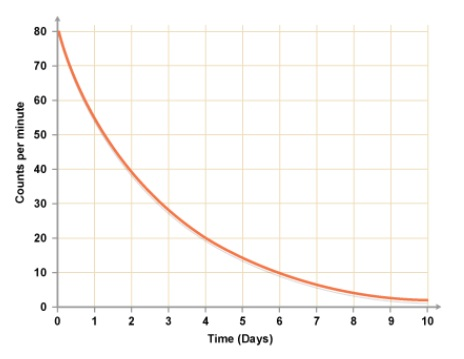Note that at time = 0, the value is No and then the value decays asymptotically toward zero.

3. Graphs like this show up in many other contexts.  For example, this looks a lot like the graph of current vs. time for a charging capacitor.  It also looks like the graph of acceleration vs. time for an object in free-fall with air resistance.  And there are a number of graphs associated with motors and generators that also have this shape.

4. When a quantity decays exponentially, in equal time intervals it will decrease by the same factor.  Most notably, the time it takes to decrease by 50% is called the “half-life”.  There is a useful formula relating the half-life to the decay constant: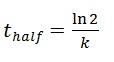Do NOT just memorize this – derive it for yourself.  If you need help getting started, you can begin by letting N(t)= ½ No.

However, all of this assumes that we already know that the function is N(t)=Noe-kt . But how do we know that?  When you see exponential decay, you should suspect that somewhere behind the scenes lurks something called a differential equation.  And that brings us (finally) to the topic of this post.

WHAT IS A DIFFERENTIAL EQUATION?

The rate at which a given radioactive sample decays is proportional to the size of the sample.”

I hope that sentence makes sense to you, intuitively.  It is saying that when there are more nuclei available to decay, the decay rate is faster.  As the sample shrinks in size, there are fewer available nuclei and so the rate slows down.

But that sentence can be re-written using mathematical symbols: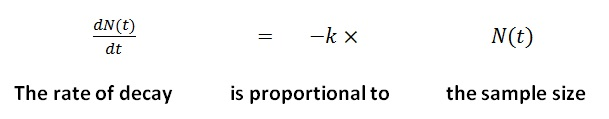The resulting equation relates a function, N(t), to one or more of its derivatives.  In this case, the highest order derivative is the first derivative so this is called a “first order differential equation”.  We are going to encounter a number of these in AP Physics and also a handful of second order differential equations as well.  You will learn how to solve some of these in math class this year.  If you continue in math and science, you may spend a number of semesters learning more about this topic.  For now, I am going to show you a method that will be sufficient for our specific needs.

WHAT IS A SOLUTION?

When you solve an algebraic equation, you find a number that you can use in place of the variable, thus obtaining a true statement.  For example, x= 3 is a solution to the equation 2x + 4 = 10 because when you replace x with 3 in that equation, you get a true result.  And even if you didn’t learn the step-by-step method of solving that equation, you could still verify that x=3 works.  It wouldn’t matter if the solution came to you in a dream!  Once you verified it, you’d know you were right.

Now we have a different kind of equation:We are not looking for a number.  We are looking for a function, one that will make a true statement when we use it to replace N(t) in that differential equation.  Here is how we are going to do this:

(Don’t worry if this doesn’t “click” at first.  We will walk through this several times.)

1. Based on our intuition, draw a graph of what the function N(t) will look like.

2. Use our extensive knowledge of pre-calculus to guess a general form of a function that has that a graph shaped like the one we just drew.

3. Take the derivative of our guess (and second derivative if needed).

4. Substitute back into the equation we are trying to solve to see if we get a true statement.

If we have guessed correctly (as we often will), we will actually pick up some bonus information.  Follow along with me and see what I mean.  We’ll start from the beginning.

We are seeking a function N(t) that will satisfy this differential equation: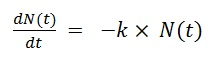1. Our intuition about radioactive decay suggests that when we find the solution, its graph, as we already noted, will look like this:2. There are a number of functions that have that shape.  For example, it could be that: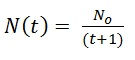It’s not a terrible guess. It has the right value at t=0 and it approaches zero asymptotically.  I don’t remember seeing that function used to model decay before and it would be all wrong for t approaching -1, but let’s try it anyway.

3. Taking the derivative, we get…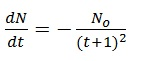4. Now, substituting the function and its derivative back into the original differential equation, we get: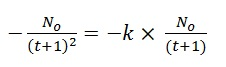Hmm…can this be true?  For a given value of k, it is true at some particular time. But we want a solution that is ALWAYS true.  There is nothing we can do to fix this one.  It turns out that our initial guess, though not terrible, was wrong.  OK, new guess.  Let’s try an exponential function.  Our memories from pre-calc  give us high hopes for this one: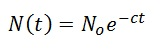In this case, we would have the derivative: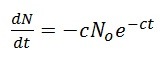(because the derivative of “e to the something” is “e to the something” and then there is that chain rule multiplier.)

But what happens when we substitute these expressions back into the original differential equation?Can this be true? Why yes, but only if c = k.  In other words, we have just learned that we had correctly guessed the general form of the solution but it can’t be just any old exponentially decaying function.  The decay constant in the function has to match the constant of proportionality in the original differential equation.  So with that adjustment, we have our solution: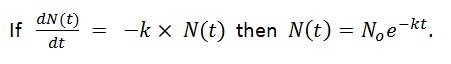This kind of thing happens a lot when we use this technique.  At the end of the process, when you ask, “can this equation be true?” you get as the answer: “yes, but only if…” followed by some new information that tells you the required value of some constant.

Closing remarks

Please do not worry if you have found this post to be challenging.  In the next practice set, I will give you a couple of additional examples and walk you through this process step-by-step.  And we will also do a couple together in class.  But review this a few times and you may realize that it is just another way to make use of derivatives and we still have not used any rules beyond the ones I showed you in the earlier posts.  But that does not mean that this was easy!

# Making the Best of Things

A Very Useful Thing About Slope Formulas

As you have worked through the previous posts and exercises, I hope you have noticed something special about places on a graph where the derivative is zero.  These places have horizontal tangent lines.  And in their own neighborhoods, they are often a local maximum or local minimum.  This piece of information is very helpful when you are trying to solve an “optimization” problem.

For example…

Suppose you are firing a projectile on a horizontal surface at a fixed launch speed, v.  And let’s also suppose that air friction is negligible.  What angle will maximize the range (horizontal distance) of the projectile?

You may remember the answer from your first year of physics.  It is intuitive and there was a way to get the answer without calculus.  But we can still use this example to illustrate the calculus method.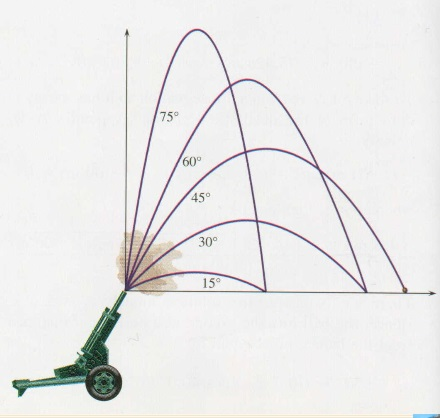(Graphic from http://outreach.phas.ubc.ca/phys420/p420_00/darren/web/range/range.html where you can also see a review of the projectile physics)

STEP 1: Express the quantity to be optimized as a function of a single variable.

In this case, a review of that website or last year’s notes will remind you that the distance varies with the launch angle by the rule: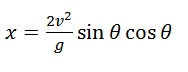In brief, we resolved the launch velocity into components, used the vertical component to determine time in air and then multiplied that time by the horizontal component of the velocity.  But now that we have this expression, for our current purposes it would be helpful to make use of the double angle identity for the sine function.  We can write: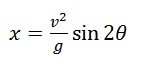Don’t worry if you would not have remembered how to do all that without notes.  The point is to learn what we can do with this expression now that we have it.

STEP 2: Find the derivative

This is one we know how to do:  we know the derivative of sine functions and we know the special case of the chain rule.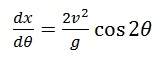STEP 3: What is the domain of interest to us?

When a math book asks for the domain of a function, they usually mean the largest, most inclusive domain possible.  For a sine function, that would be all real numbers.  But that is not what we are after here.  We need to think about the portion of the domain that is relevant.  To fire this cannonball as far as we can, clearly we will choose an angle between 0 and π/2 radians.

STEP 4: Where in that domain is the derivative equal to zero?

In this case…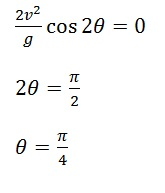(OK, call it 45 degrees if you prefer.)

STEP 5: (Your math teacher may not like this) Convince ourselves that we are done.

In math class, once you have found where the derivative is zero (or undefined), you are not nearly done.  You have to investigate each of these “critical points” to see what kind of extreme it is and to justify your conclusion with such things as sign charts and 2nd derivative tests.  But in physics class, you are going to get away a little easier because of the following claim:

If a “smooth” function has only one critical point on some interval and the value of the function is higher at that critical point than at either of the endpoints, then that value is the “absolute maximum” value.

So in the example at hand, we know the cannonball’s range is zero when the angle is zero, and its zero when the angle is 90 degrees (as the ball goes straight up and down).  But it is NOT zero when the launch angle is 45 degrees.  But the tangent line is horizontal at 45 degrees.  So that’s the “best” angle.

You still have to learn to do things the math-class way.  There are lots of functions with multiple critical points.  And there are functions that have horizontal tangent lines at places that turn out not to maxima or minima.  So you do have to know how to investigate further.

But the problems you will see in AP Physics don’t turn out that way.  Most often, there will be only one critical point and it will be the one we were looking for.  Here is another example I have always liked.Suppose you have a large cylindrical tank full of water, open at the top.  You are going to punch a hole in the tank somewhere along its side and you want the resulting jet of water to land as far from the tank as possible.  Where should you make the hole?  And where will that initial jet of water land?

Let’s say you make the hole y units from the ground and the initial jet lands x units from the tank.

STEP 1: Determine x as a function of y

First we need the “speed of efflux” which you can think of as the horizontal launch velocity of the water coming out of the hole.  There is a neat result from fluid dynamics that shows that the water leaves the tank at the same speed as if it had free-fallen from the top of the tank.  It’s called Torricielli’s Law.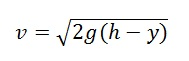http://en.wikipedia.org/wiki/Torricelli’s_law  (where I also got the graphic above)

Now, we just find the time in air and multiply by that launch speed.

For time in air, we can use the fact that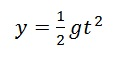and solve for time to get: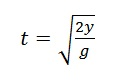So for the distance as a function of the height of the hole we get: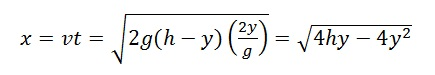STEP 2: Find the derivative

Well, it seems we need the more advanced version of the chain rule. But there is a trick to make things easier.  The distance x will be at its maximum when the term inside the square root is at its maximum.  So let’s ignore the square root and just maximize the innards.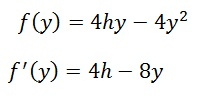STEP 3: Domain of interest?  We can’t make a hole below the ground or above the height of the cylinder so we only have to consider y values between 0 and h.

STEP 4: Set the derivative equal to zeroWe need a hole in the middle!

STEP 5:  Convince ourselves that we are done

There was only one critical point.  And the endpoints both lead to a distance of zero.  So the hole at y=h/2 is the one that gives us the farthest landing point.

And what is that landing point? Substitute y=h/2 into our original function, which was:I will leave the substitution and clean-up to you.  I will say that it cleans up quite nicely and has a memorable result.  The first of my students to post the correct result in a comment wins some silly thing or other.

Quick Recap and Closing Remarks

In AP Physics, when you want to find the maximum or minimum possible value of some quantity, most of the time it will just be a matter of taking a derivative, then setting it equal to zero and then waving your hands around to convince us that you are done.

The hardest part of the process is not going to be the calculus.  It’s going to be setting up the variables and finding the expression for the quantity you hope to optimize.  That’s why you had all those homework problems back in pre-calc that said “Express this thing in terms of that thing”.

I guess I should mention that if you are having trouble either finding the derivative or solving after you set it equal to zero, you may find your TI82 to be of some use.  But honestly, sometimes it feels like the calculator only gives intelligible answers to people who already know what the solution looks like.